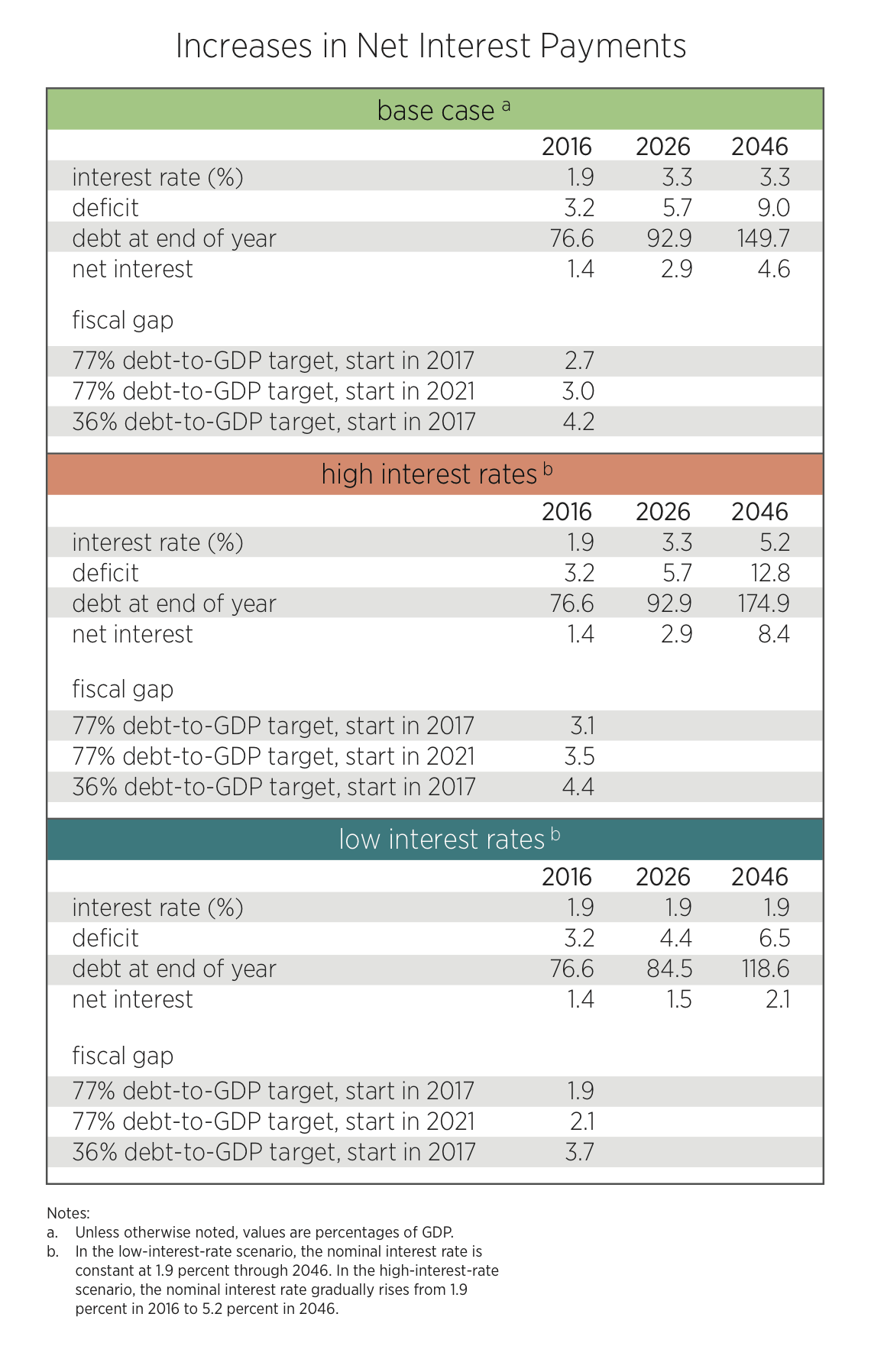### Low Interest Rates and the Economy: A Mercatus Colloquium

December 12, 2016

## Interest Rates and the Federal Budget Outlook

Series Information

This essay is the tenth in a twelve-essay colloquium on the effect of low interest rates on the economy. To read other essays in the series, click here.

Over the past decade, interest rates on government debt have remained remarkably low by historical standards, with some countries even venturing into negative-rate territory. Although policymakers and researchers are still trying to understand the reasons for and implications of low interest rates, they are also concerned about the likelihood and effects of potential future increases in interest rates.

In this paper, we focus on the implications of different interest rate scenarios for federal budget projections over a medium-term (10- to 30-year) horizon. We use the methodology and assumptions reported in Auerbach and Gale (2016), which has been updated to reflect the most recent projections by government agencies.

Our baseline medium-term projections indicate that federal debt will rise from 77 percent of GDP at the end of 2016 to 93 percent of GDP in 2026 and all the way up to 150 percent of GDP by 2046 (see table 1 and figure 1). Those projections have increases in interest rates already built in. We use interest rate assumptions developed by the Congressional Budget Office (CBO), which projects that the average nominal interest rate on federal debt will rise from 1.9 percent in 2016 to 3.3 percent in 2026. The CBO expects inflation to remain fairly constant, so the projections simply increase in real interest rates through 2026. We then hold the interest rate constant at 3.3 percent through 2046. Because of rising interest rates and already high levels of debt, net interest payments rise from 1.4 percent of GDP in 2016 to 4.6 percent of GDP in 2046 (table 1). By comparison, the previous high for net interest payments as a share of GDP was 3.2 percent in 1991.The debt-to-GDP projections are sensitive to interest rate assumptions. Lower interest rates reduce the projected medium-term increase in debt. For example, if the interest rate on federal debt stays at its current low of 1.9 percent for the next 30 years, the debt-to-GDP ratio would still rise over time but only to 119 percent of GDP in 2046 (table 1, figure 1). Net interest payments would also rise—because debt rises—but only to 2.1 percent of GDP in 2046.

But if interest rates rise faster than in the base case, the debt-to-GDP ratio will grow more quickly. Suppose interest rates rise as the CBO projects through 2026 and then gradually rise further to 5.2 percent by 2046. The latter figure represents the long-term nominal interest rate projected by the Social Security trustees, adjusted for differences in economic growth assumptions between the trustees’ report and the CBO Long-Term Budget Outlook. (Economic growth from 2026 to 2046 averages 4.43 percent in the trustees’ report and 4.25 percent in the Long-Term Budget Outlook.) Under those assumptions, the debt-to-GDP ratio would reach 175 percent by 2046 (figure 1), with net interest payments equaling 8.4 percent of GDP in that year (table 1).

The “fiscal gap” measures the size of the constant-share-of-GDP increase in taxes and the reductions in noninterest spending that would be required to meet a given future debt to GDP target in a given year. Under the base case for interest rates, with policy changes starting in 2017, the fiscal gap to reach the current debt to GDP ratio of 77 percent in 2046 is 2.7 percent of GDP (table 1).

Changes in interest rates have larger effects on the projected debt to GDP ratio than on the fiscal gap, because debt increases affect the fiscal gap only to the extent of the added annual interest payments (as a share of GDP) that are needed to keep the debt-to-GDP ratio from growing. Under the low-interest-rate scenario, the fiscal gap is 1.9 percent of GDP, while under the high-interest-rate scenario, it is 3.1 percent of GDP (compared to 2.7 percent of GDP in the base case).

Thus, even if interest rate rates stay low, a sizable change in noninterest spending, in taxes, or in both is still necessary to attain a debt-to-GDP ratio of 77 percent in 30 years. The fiscal gap is larger to the extent that policy adjustments begin at a date later than 2017 or the debt target is reduced below the current 77 percent level (table 1).

Although higher interest rates make the medium-term situation worse, they also increase the likelihood that policymakers will respond. When policymakers reached agreement on the 1990 and 1993 budget deals, the debt was much lower than it is today, but net interest payments as a share of GDP were higher. The amount of funds going to net interest payments helped galvanize attention to the deficit problem at that time.